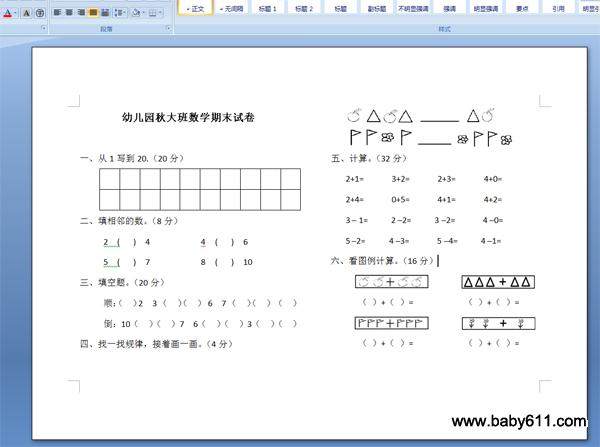幼儿园秋大班数学期末试卷幼儿园秋大班数学期末试卷

一、从1写到20.（20分）

二、填相邻的数。（8分）

2  (   )  4           4  (   )  6

5  (   )  7           8  (   )  10

三、填空题。（20分）

顺：（  ）2  3 （  ）（  ） 6  7 （  ）（  ） （  ）

倒：10（  ）（  ）7  6（  ）（  ）3（  ）（  ）

四、找一找规律，接着画一画。（4分）

五、计算。（32分）

2+1=      3+2=      2+3=      4+0=

2+4=      0+5=      4+1=      4+2=

3 - 1=     2 -2=     3 -2=      4 -0=

5 -2=     4 -3=      5 -4=     4 -1=

六、看图例计算。（16分）

（ ）+（ ）=           （ ）+（ ）=

（ ）+（ ）=           （ ）+（ ）=

• 幼儿园试卷分类
• 将此试卷分享到：
• 大班试卷最新更新